Search by Topic

Resources tagged with Physics similar to Stonehenge:

Filter by: Content type:
Age range:
Challenge level:

There are 77 results

Broad Topics > Applications > PhysicsSweeping Satellite

Age 16 to 18 Challenge Level:

Derive an equation which describes satellite dynamics.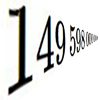Big and Small Numbers in Physics - Group Task

Age 16 to 18 Challenge Level:

Work in groups to try to create the best approximations to these physical quantities.Moving Stonehenge

Age 16 to 18 Challenge Level:

A look at the fluid mechanics questions that are raised by the Stonehenge 'bluestones'.Mach Attack

Age 16 to 18 Challenge Level:

Have you got the Mach knack? Discover the mathematics behind exceeding the sound barrier.Levels of Bohr

Age 16 to 18 Challenge Level:

Look at the units in the expression for the energy levels of the electrons in a hydrogen atom according to the Bohr model.The Lorentz Force Law

Age 16 to 18 Challenge Level:

Explore the Lorentz force law for charges moving in different ways.Ideal Gases

Age 16 to 18 Challenge Level:

Problems which make you think about the kinetic ideas underlying the ideal gas laws.The Not-so-simple Pendulum 1

Age 16 to 18 Challenge Level:

See how the motion of the simple pendulum is not-so-simple after all.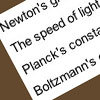Universal Time, Mass, Length

Age 16 to 18 Short Challenge Level:

Can you work out the natural time scale for the universe?Guessing the Graph

Age 14 to 16 Challenge Level:

Can you suggest a curve to fit some experimental data? Can you work out where the data might have come from?Engnrich

Age 16 to 18 Challenge Level:

engNRICH is the area of the stemNRICH Advanced site devoted to the mathematics underlying the study of engineeringPhysnrich

Age 14 to 18 Challenge Level:

PhysNRICH is the area of the StemNRICH site devoted to the mathematics underlying the study of physicsChemnrich

Age 14 to 18 Challenge Level:

chemNRICH is the area of the stemNRICH site devoted to the mathematics underlying the study of chemistry, designed to help develop the mathematics required to get the most from your study. . . .Ramping it Up

Age 16 to 18 Challenge Level:

Look at the calculus behind the simple act of a car going over a step.Core Scientific Mathematics

Age 14 to 18 Challenge Level:

This is the area of the advanced stemNRICH site devoted to the core applied mathematics underlying the sciences.Neural Nets

Age 16 to 18

Find out some of the mathematics behind neural networks.Drug Stabiliser

Age 16 to 18 Challenge Level:

How does the half-life of a drug affect the build up of medication in the body over time?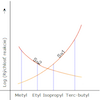Reaction Types

Age 16 to 18 Challenge Level:

Explore the rates of growth of the sorts of simple polynomials often used in mathematical modelling.Whose Line Graph Is it Anyway?

Age 16 to 18 Challenge Level:

Which line graph, equations and physical processes go together?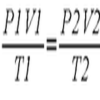Ideal Axes

Age 16 to 18 Challenge Level:

Explore how can changing the axes for a plot of an equation can lead to different shaped graphs emerging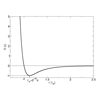Lennard Jones Potential

Age 16 to 18 Challenge Level:

Investigate why the Lennard-Jones potential gives a good approximate explanation for the behaviour of atoms at close ranges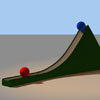Whoosh

Age 16 to 18 Challenge Level:

A ball whooshes down a slide and hits another ball which flies off the slide horizontally as a projectile. How far does it go?Escape from Planet Earth

Age 16 to 18 Challenge Level:

How fast would you have to throw a ball upwards so that it would never land?Cannon Balls

Age 16 to 18 Short Challenge Level:

How high will a ball taking a million seconds to fall travel?Approximately Certain

Age 14 to 18 Challenge Level:

Estimate these curious quantities sufficiently accurately that you can rank them in order of sizeGravity Paths

Age 16 to 18 Challenge Level:

Where will the spaceman go when he falls through these strange planetary systems?Striking Gold

Age 16 to 18 Challenge Level:

Investigate some of the issues raised by Geiger and Marsden's famous scattering experiment in which they fired alpha particles at a sheet of gold.Lunar Leaper

Age 16 to 18 Challenge Level:

Gravity on the Moon is about 1/6th that on the Earth. A pole-vaulter 2 metres tall can clear a 5 metres pole on the Earth. How high a pole could he clear on the Moon?Go Spaceship Go

Age 16 to 18 Challenge Level:

Show that even a very powerful spaceship would eventually run out of overtaking powerA Question of Scale

Age 14 to 16 Challenge Level:

Use your skill and knowledge to place various scientific lengths in order of size. Can you judge the length of objects with sizes ranging from 1 Angstrom to 1 million km with no wrong attempts?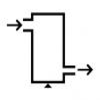Keep Your Momentum Going

Age 16 to 18 Challenge Level:

A look at a fluid mechanics technique called the Steady Flow Momentum Equation.Pumping the Power

Age 16 to 18 Challenge Level:

What is an AC voltage? How much power does an AC power source supply?New Units for Old

Age 16 to 18 Challenge Level:

Can you match up the entries from this table of units?The Power of Dimensional Analysis

Age 14 to 18

An introduction to a useful tool to check the validity of an equation.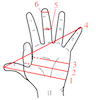Age 14 to 16 Challenge Level:

Which units would you choose best to fit these situations?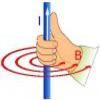Electromagnetism

Age 16 to 18

Read all about electromagnetism in our interactive article.Eudiometry

Age 16 to 18 Challenge Level:

When a mixture of gases burn, will the volume change?Which Twin Is Older?

Age 16 to 18

A simplified account of special relativity and the twins paradox.Stemnrich - Technology

Age 11 to 16 Challenge Level:

This is the technology section of stemNRICH - Core.The Ultra Particle

Age 16 to 18 Challenge Level:

Explore the energy of this incredibly energetic particle which struck Earth on October 15th 1991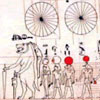Ancient Astronomical Terms

Age 11 to 18

Some explanations of basic terms and some phenomena discovered by ancient astronomersPack Man

Age 16 to 18 Challenge Level:

A look at different crystal lattice structures, and how they relate to structural propertiesModelling Assumptions in Mechanics

Age 16 to 18

An article demonstrating mathematically how various physical modelling assumptions affect the solution to the seemingly simple problem of the projectile.Motorbike Momentum

Age 16 to 18 Challenge Level:

A think about the physics of a motorbike riding upside downBattery Modelling

Age 16 to 18 Challenge Level:

Find out how to model a battery mathematicallyMaths in the Undergraduate Physical Sciences

Age 16 to 18

An article about the kind of maths a first year undergraduate in physics, engineering and other physical sciences courses might encounter. The aim is to highlight the link between particular maths. . . .The Real Hydrogen Atom

Age 16 to 18 Challenge Level:

Dip your toe into the world of quantum mechanics by looking at the Schrodinger equation for hydrogen atoms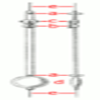The Not-so-simple Pendulum 2

Age 16 to 18 Challenge Level:

Things are roughened up and friction is now added to the approximate simple pendulumPowerfully Fast

Age 16 to 18 Challenge Level:

Explore the power of aeroplanes, spaceships and horses.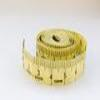Bigger or Smaller?

Age 14 to 16 Challenge Level:

When you change the units, do the numbers get bigger or smaller?